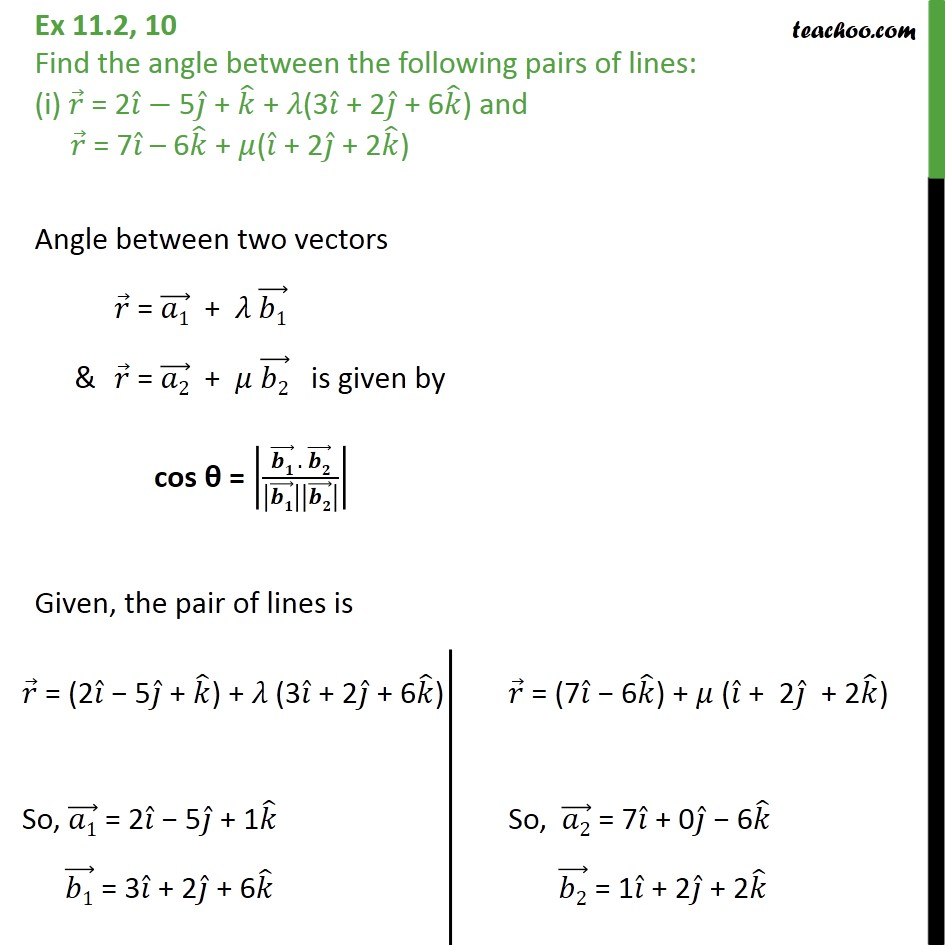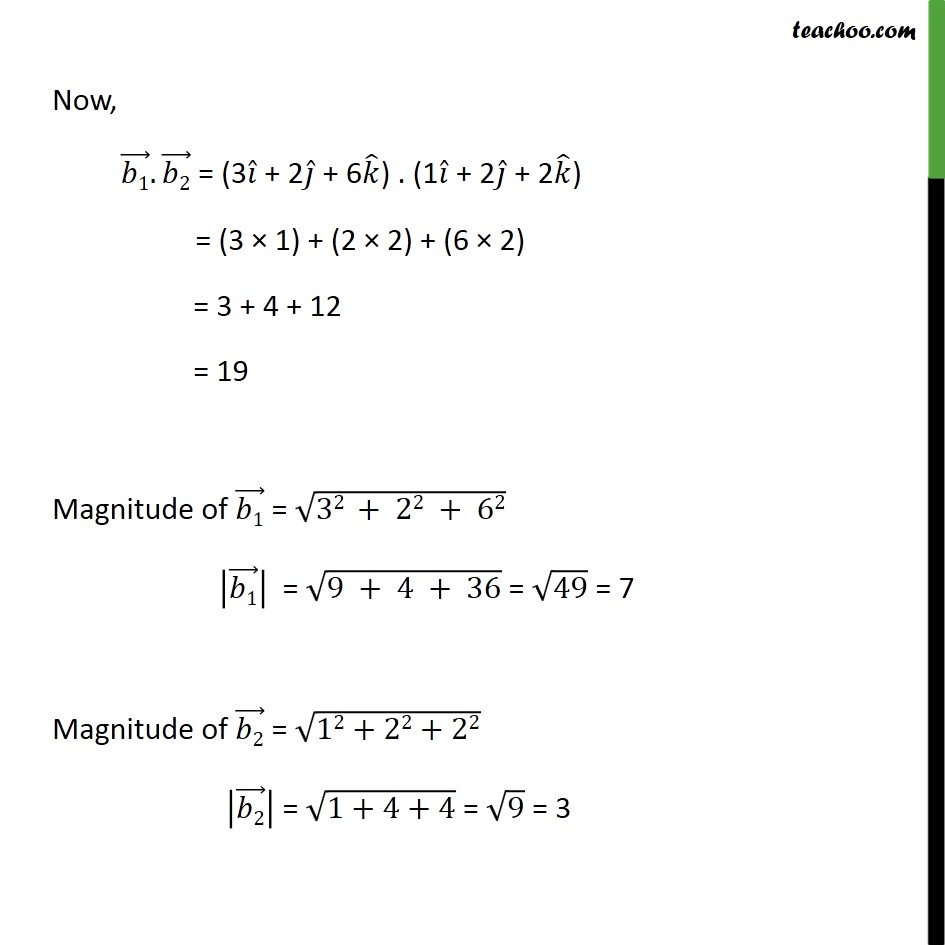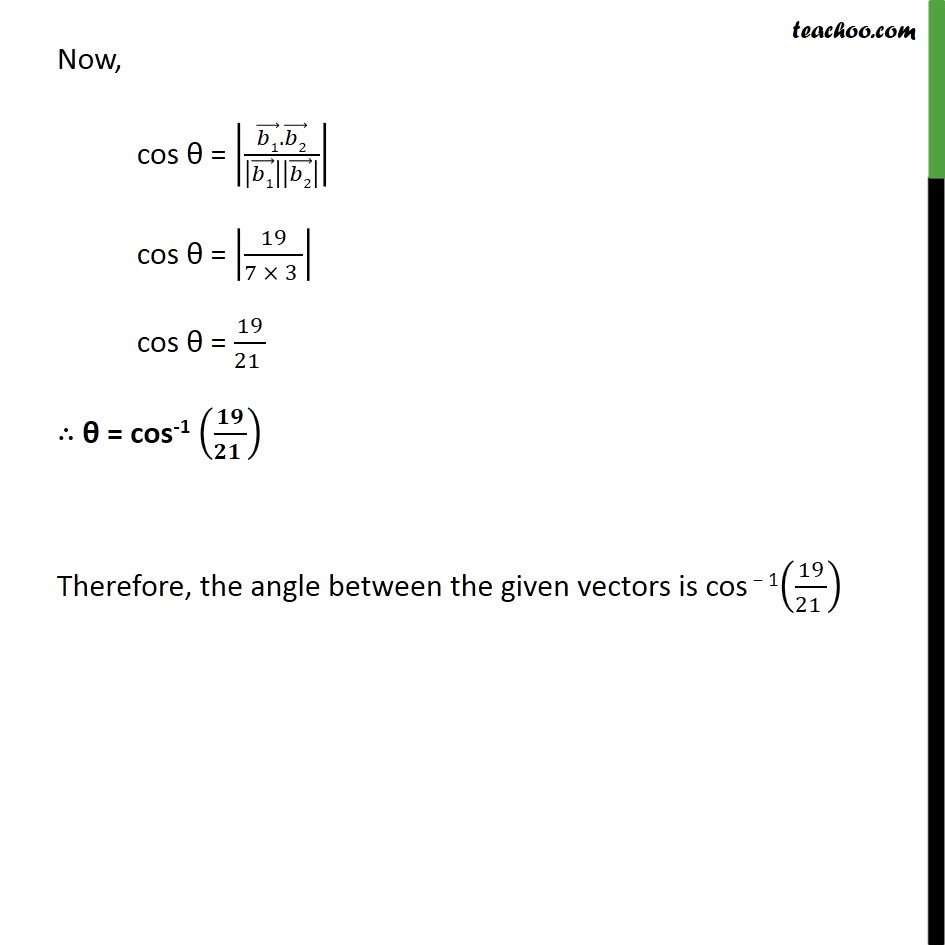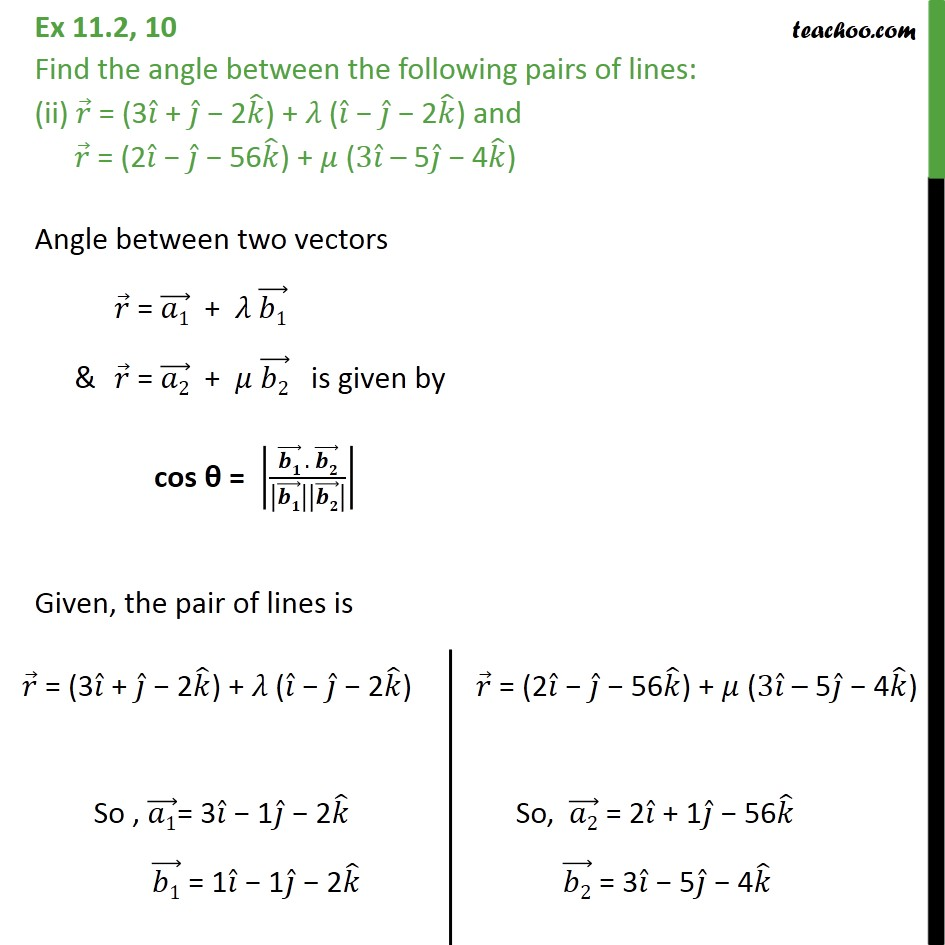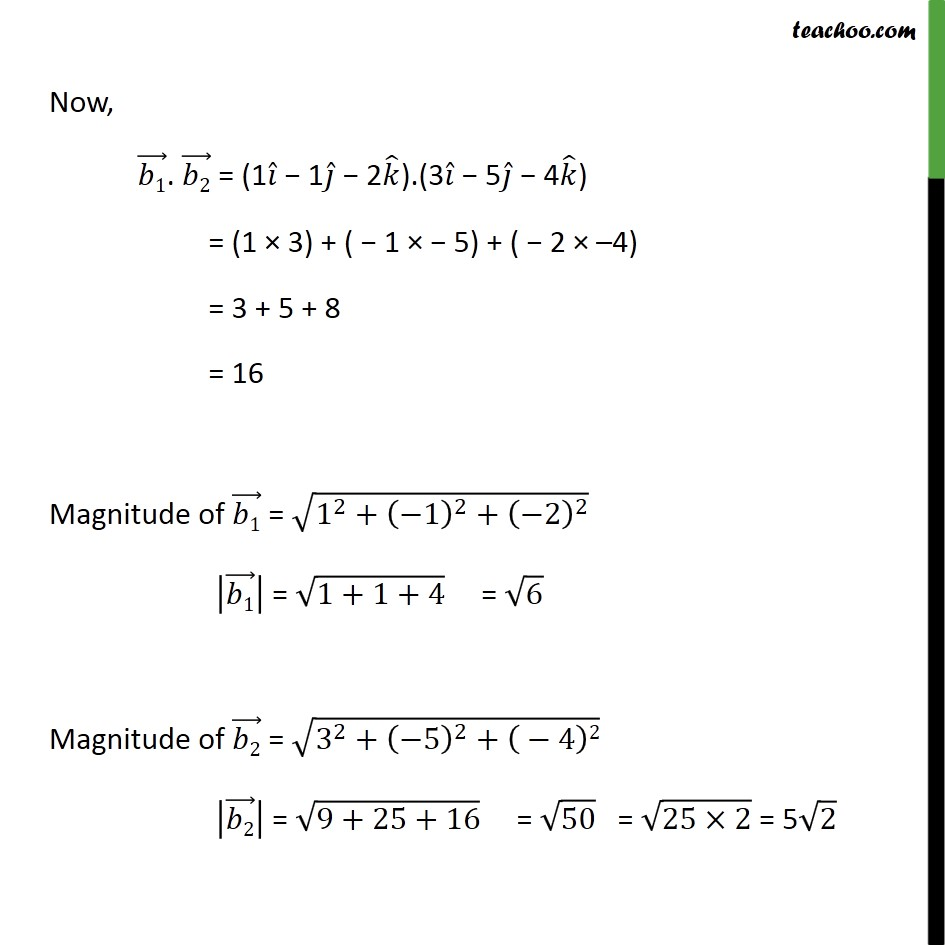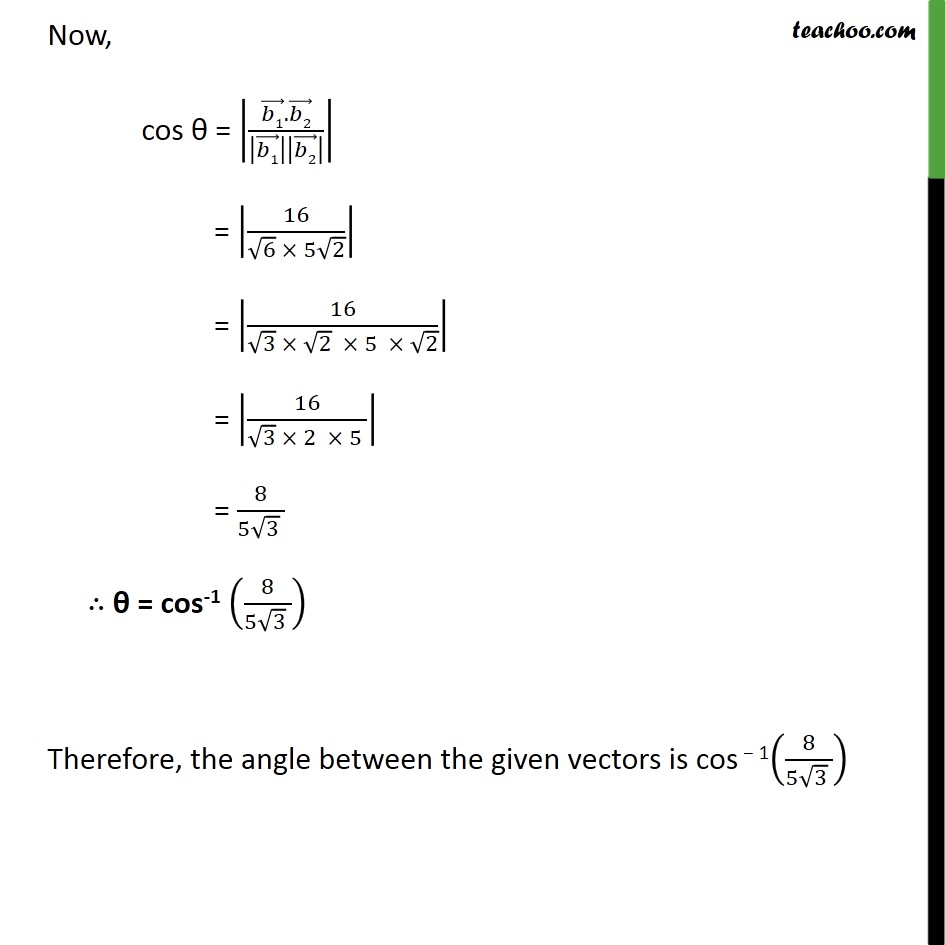1. Chapter 11 Class 12 Three Dimensional Geometry
2. Serial order wise
3. Ex 11.2

Transcript

Ex 11.2, 10 Find the angle between the following pairs of lines: (i) = 2 5 + + (3 + 2 + 6 ) and = 7 6 + ( + 2 + 2 ) Angle between two vectors = 1 + 1 & = 2 + 2 is given by cos = . Given, the pair of lines is Now, 1 . 2 = (3 + 2 + 6 ) . (1 + 2 + 2 ) = (3 1) + (2 2) + (6 2) = 3 + 4 + 12 = 19 Magnitude of 1 = 32 + 22 + 62 1 = 9 + 4 + 36 = 49 = 7 Magnitude of 2 = 12+22+22 2 = 1+4+4 = 9 = 3 Now, cos = 1 . 2 1 2 cos = 19 7 3 cos = 19 21 = cos-1 Therefore, the angle between the given vectors is cos 1 19 21 Ex 11.2, 10 Find the angle between the following pairs of lines: (ii) = (3 + 2 ) + ( 2 ) and = (2 56 ) + (3 5 4 ) Angle between two vectors = 1 + 1 & = 2 + 2 is given by cos = . Given, the pair of lines is Now, 1 . 2 = (1 1 2 ).(3 5 4 ) = (1 3) + ( 1 5) + ( 2 4) = 3 + 5 + 8 = 16 Magnitude of 1 = 1 2 + 1 2 + 2 2 1 = 1+1+4 = 6 Magnitude of 2 = 3 2 + 5 2 + 4 2 2 = 9+25+16 = 50 = 25 2 = 5 2 Now, cos = 1 . 2 1 2 = 16 6 5 2 = 16 3 2 5 2 = 16 3 2 5 = 8 5 3 = cos-1 8 5 3 Therefore, the angle between the given vectors is cos 1 8 5 3

Ex 11.2